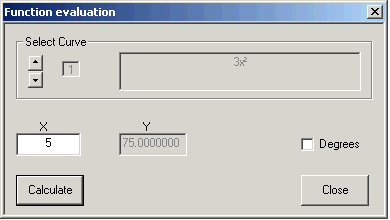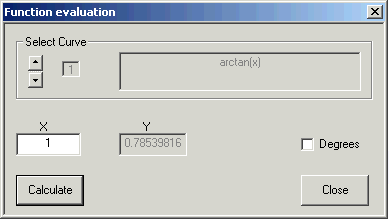# Evaluate

Suppose you have drawn the graph of a function, and you need its value for some value of x.

Click Evaluate and then  Get Y Value  at the top of the window. This window opens:Type the x value into the X box, and click on Calculate.

I put x = 5 into the function  3x2 and got  y = 75. And it's right square five to get 25, and 3 times 25 is 75.

If you have several curve formulas entered, use Select Curve to tell CalGraph which one to calculate. The arrows step through the formulas you have put in, and you see them in the box on the right side.

For trigonometry, CalGraph usually works in radians. If you want to calculate sine, cosine or tangent  using degrees, check the degrees box. Your graph will not change, but in the Function evaluation window  y will be evaluated treating the number inside the trig function as degrees.

Notice that inverse trig functions (sin-1, cos-1 and tan-1) are always in radians. To get the angle in degrees, multiply the result of the inverse trig by 180 and divide by pi.  Here's one.The tan-1 of 1 is 0.78539816. Multiply 0.78539816 by 180 and divide by pi, and you get 44.9999998°, which is pretty close to 45°. (arctan is another name for writing tan-1)

If you have no curve formula entered, CalGraph can't calculate anything.

Another way to find a value at a point on a graph is to click that point. The x and y values of that point appear in the lower left corner of the CalGraph window. Of course, you might not have clicked exactly where you want.